# Multilabel Image Classification Using Deep Learning

This example shows how to use transfer learning to train a deep learning model for multilabel image classification.

In binary or multiclass classification, a deep learning model classifies images as belonging to one of two or more classes. The data used to train the network often contains clear and focused images, with a single item in frame and without background noise or clutter. This data is often not an accurate representation of the type of data the network will receive during deployment. Additionally, binary and multiclass classification can apply only a single label to each image, leading to incorrect or misleading labeling.

In this example, you train a deep learning model for multilabel image classification by using the COCO data set, which is a realistic data set containing objects in their natural environments. The COCO images have multiple labels, so an image depicting a dog and a cat has two labels.

In multilabel classification, in contrast to binary and multiclass classification, the deep learning model predicts the probability of each class. The model has multiple independent binary classifiers, one for each class—for example, "Cat" and "Not Cat" and "Dog" and "Not Dog."Load a pretrained ResNet-50 network. If the Deep Learning Toolbox Model for ResNet-50 Network support package is not installed, then the software provides a download link. ResNet-50 is trained on more than a million images and can classify images into 1000 object categories, such as keyboard, mouse, pencil, and many animals. This example uses transfer learning to retrain a ResNet-50 pretrained network for multilabel classification.

Load the pretrained network and extract the image input size.

```net = resnet50; inputSize = net.Layers(1).InputSize;```

### Prepare Data

Download and extract the COCO 2017 training and validation images and their labels from https://cocodataset.org/#download by clicking the "2017 Train images", "2017 Val images", and "2017 Train/Val annotations" links. Save the data in a folder named `"COCO"`. The COCO 2017 data set was collected by Coco Consortium. Depending on your internet connection, the download process can take time.

Train the network on a subset of the COCO data set. For this example, train the network to recognize 12 different categories: dog, cat, bird, horse, sheep, cow, bear, giraffe, zebra, elephant, potted plant, and couch.

```categoriesTrain = ["dog" "cat" "bird" "horse" "sheep" "cow" "bear" "giraffe" "zebra" "elephant" "potted plant" "couch"]; numClasses = length(categoriesTrain);```

Specify the location of the training data.

```dataFolder = fullfile(tempdir,"COCO"); labelLocationTrain = fullfile(dataFolder,"annotations_trainval2017","annotations","instances_train2017.json"); imageLocationTrain = fullfile(dataFolder,"train2017");```

Use the supporting function `prepareData`, defined at the end of this example, to prepare the data for training.

1. Extract the labels from the file `labelLocationTrain` using the `jsondecode` function.

2. Find the images that belong to the classes of interest.

3. Find the number of unique images. Many images have more than one of the class labels and, therefore, appear in the image lists for multiple categories.

4. Create the one-hot encoded category labels by comparing the image ID with the lists of image IDs for each category.

5. Create an augmented image datastore containing the images and an image augmentation scheme.

```[dataTrain,encodedLabelTrain] = prepareData(labelLocationTrain,imageLocationTrain,categoriesTrain,inputSize,true); numObservations = dataTrain.NumObservations```
```numObservations = 30492 ```

The training data contains 30,492 images from 12 classes. Each image has a binary label that indicates whether it belongs to each of the 12 classes.

Prepare the validation data in the same way as the training data.

```labelLocationVal = fullfile(dataFolder,"annotations_trainval2017","annotations","instances_val2017.json"); imageLocationVal = fullfile(dataFolder,"val2017"); [dataVal,encodedLabelVal] = prepareData(labelLocationVal,imageLocationVal,categoriesTrain,inputSize,false);```

### Inspect Data

View the number of labels for each class.

```numObservationsPerClass = sum(encodedLabelTrain,1); figure bar(numObservationsPerClass) ylabel("Number of Observations") xticklabels(categoriesTrain)```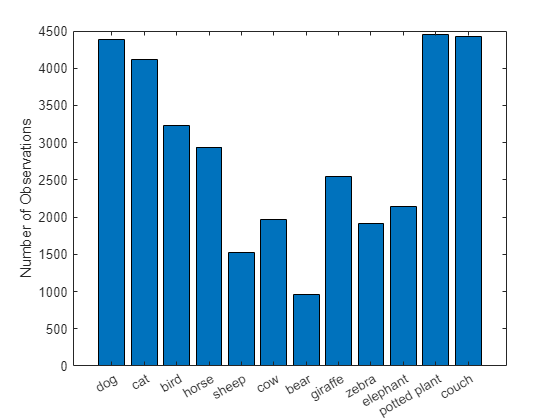View the average number of labels per image.

```numLabelsPerObservation = sum(encodedLabelTrain,2); mean(numLabelsPerObservation)```
```ans = 1.1352 ```
```figure histogram(numLabelsPerObservation) hold on ylabel("Number of Observations") xlabel("Number of Labels") hold off```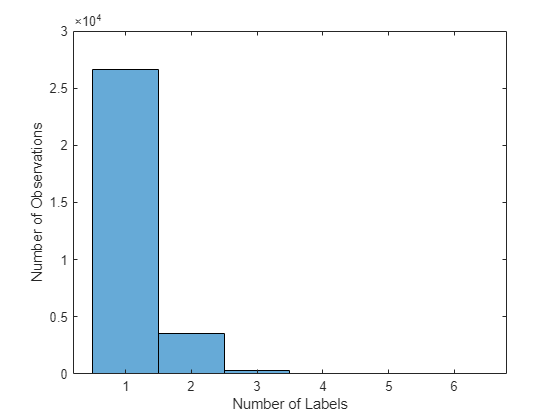### Adapt Pretrained Network for Transfer Learning

The final layers of the network contain information on how to combine the features that the network extracts into probabilities, a loss value, and predicted labels. These layers are currently defined for a single label classification task with 1000 classes. You can easily adapt this network to a multilabel classification task by replacing the last learnable layer, the softmax layer, and the classification layer. You can adapt this network programmatically or interactively using Deep Network Designer.

`lgraph = layerGraph(net);`

#### Replace Last Learnable Layer

The final fully connected layer of the network is configured for 1000 classes. To adapt the network to classify images into 12 classes, replace the final fully connected layer with a new layer adapted to the new data set. Set the output size to match the number of classes in the new data. To make learning faster in the new layers than in the transferred layers, increase the `WeightLearnRateFactor` and the `BiasLearnRateFactor` values of the new layer.

```newLearnableLayer = fullyConnectedLayer(numClasses, ... Name="new_fc", ... WeightLearnRateFactor=10, ... BiasLearnRateFactor=10); lgraph = replaceLayer(lgraph,"fc1000",newLearnableLayer);```

#### Replace Softmax Layer

For single label classification, the network has a softmax layer followed by a classification output layer. The softmax layer computes the scores for each label, where the scores sum to 1. The highest score is the predicted class for that input. To adapt this network for multilabel classification, you must replace the softmax layer with a sigmoid layer. The sigmoid layer produces independent probabilities for each class. You can use these probabilities to predict multiple labels for a single input image.

```newActivationLayer = sigmoidLayer(Name="sigmoid"); lgraph = replaceLayer(lgraph,"fc1000_softmax",newActivationLayer);```

#### Replace Output Layer

Finally, replace the output layer with a custom binary cross-entropy loss output layer. The binary cross-entropy loss layer computes the loss between the target labels and the predicted labels. This layer is attached as the supporting file `CustomBinaryCrossEntropyLossLayer.m`. To access this file, open this example as a live script.

```newOutputLayer = CustomBinaryCrossEntropyLossLayer("new_classoutput"); lgraph = replaceLayer(lgraph,"ClassificationLayer_fc1000",newOutputLayer);```

The network is now ready to train.

### Training Options

Specify the options to use for training. Train using an SGDM solver with an initial learning rate of 0.0005. Set the mini-batch size to 32 and train for a maximum of 10 epochs. Specify the validation data and set training to stop once the validation loss fails to decrease for five consecutive evaluations.

```options = trainingOptions("sgdm", ... InitialLearnRate=0.0005, ... MiniBatchSize=32, ... MaxEpochs=10, ... Verbose= false, ... ValidationData=dataVal, ... ValidationFrequency=100, ... ValidationPatience=5, ... Plots="training-progress");```

### Train Network

To save time while running this example, load a trained network by setting `doTraining` to `false`. To train the network yourself, set `doTraining` to `true`.

The custom binary cross-entropy loss layer inherits from the `nnet.layer.RegressionLayer` class. Therefore, the training plot displays the RMSE and the loss. For this example, the loss is a more useful measure of network performance.

```doTraining = false; if doTraining trainedNet = trainNetwork(dataTrain,lgraph,options); else filename = matlab.internal.examples.downloadSupportFile('nnet', ... 'data/multilabelImageClassificationNetwork.zip'); filepath = fileparts(filename); dataFolder = fullfile(filepath,'multilabelImageClassificationNetwork'); unzip(filename,dataFolder); load(fullfile(dataFolder,'multilabelImageClassificationNetwork.mat')); end```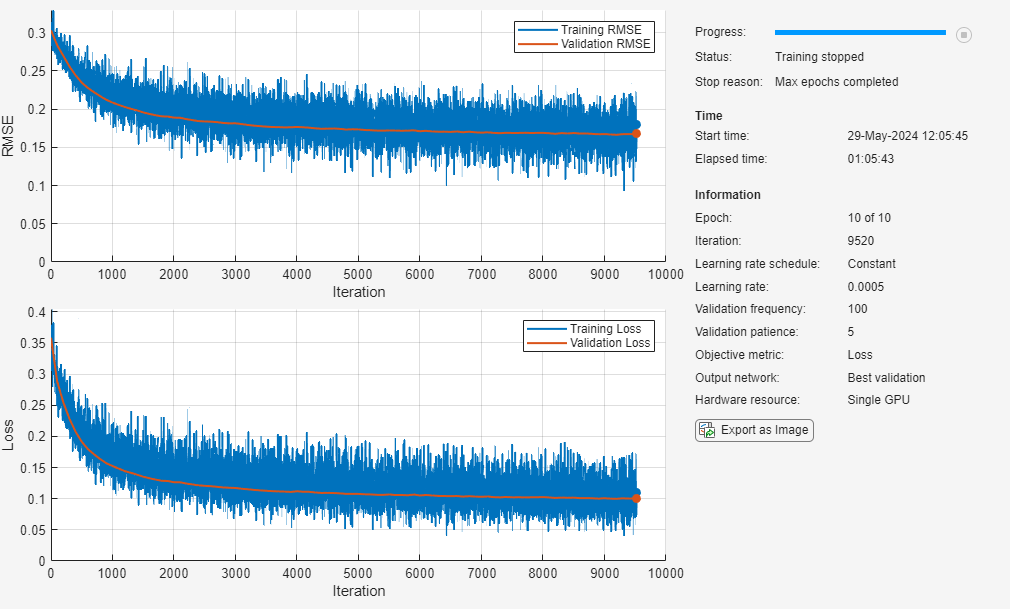### Assess Model Performance

Assess the model performance on the validation data.

The model predicts the probability of each class being present in the input image. To use these probabilities to predict the classes of the image, you must define a threshold value. The model predicts that the image contains the classes with probabilities that exceed the threshold.

The threshold value controls the rate of false positives versus false negatives. Increasing the threshold reduces the number of false positives, whereas decreasing the threshold reduces the number of false negatives. Different applications will require different threshold values. For this example, set a threshold value of 0.5.

`thresholdValue = 0.5;`

Use the `predict` function to compute the class scores for the validation data.

`scores = predict(trainedNet,dataVal);`

Convert the scores to a set of predicted classes using the threshold value.

`YPred = double(scores >= thresholdValue);`

#### F1-score

Two common metrics for accessing model performance are precision (also known as the positive predictive value) and recall (also known as sensitivity).

`$\mathrm{Precision}=\frac{\mathrm{True}\text{\hspace{0.17em}}\mathrm{Positive}}{\mathrm{True}\text{\hspace{0.17em}}\mathrm{Positive}+\mathrm{False}\text{\hspace{0.17em}}\mathrm{Postive}}\text{\hspace{0.17em}}$`

`$\mathrm{Recall}=\frac{\mathrm{True}\text{\hspace{0.17em}}\mathrm{Positive}}{\mathrm{True}\text{\hspace{0.17em}}\mathrm{Positive}+\mathrm{False}\text{\hspace{0.17em}}\mathrm{Negative}}\text{\hspace{0.17em}}$`

For multilabel tasks, you can calculate the precision and recall for each class independently and then take the average (known as macro-averaging) or you can calculate the global number of true positives, false positives, and false negatives and use those values to calculate the overall precision and recall (known as micro-averaging). Throughout this example, use the micro-precision and the micro-recall values.

To combine the precision and recall into a single metric, compute the F1-score . The F1-score is commonly used for evaluating model accuracy.

`${F}_{1}=2\left(\frac{precision*recall}{precision+recall}\right)$`

A value of 1 indicates that the model performs well. Use the supporting function `F1Score` to compute the micro-average F1-score for the validation data.

`FScore = F1Score(encodedLabelVal,YPred)`
```FScore = 0.8158 ```

#### Jaccard Index

Another useful metric for assessing performance is the Jaccard index, also known as intersection over union. This metric compares the proportion of correct labels to the total number of labels. Use the supporting function `jaccardIndex` to compute the Jaccard index for the validation data.

`jaccardScore = jaccardIndex(encodedLabelVal,YPred)`
```jaccardScore = 0.7092 ```

#### Confusion Matrix

To investigate performance at the class level, for each class, compute the confusion chart using the predicted and true binary labels.

```figure tiledlayout("flow") for i = 1:numClasses nexttile confusionchart(encodedLabelVal(:,i),YPred(:,i)); title(categoriesTrain(i)) end```### Investigate Threshold Value

Investigate how the threshold value impacts the model assessment metrics. Calculate the F1-score and the Jaccard index for different threshold values. Additionally, use the supporting function `performanceMetrics` to calculate the precision and recall for different threshold values.

```thresholdRange = 0.1:0.1:0.9; metricsName = ["F1-score","Jaccard Index","Precision","Recall"]; metrics = zeros(4,length(thresholdRange)); for i = 1:length(thresholdRange) YPred = double(scores >= thresholdRange(i)); metrics(1,i) = F1Score(encodedLabelVal,YPred); metrics(2,i) = jaccardIndex(encodedLabelVal,YPred); [precision, recall] = performanceMetrics(encodedLabelVal,YPred); metrics(3,i) = precision; metrics(4,i) = recall; end```

Plot the results.

```figure tiledlayout("flow") for i = 1:4 nexttile plot(thresholdRange,metrics(i,:),"-*") title(metricsName(i)) xlabel("Threshold") ylabel("Score") end```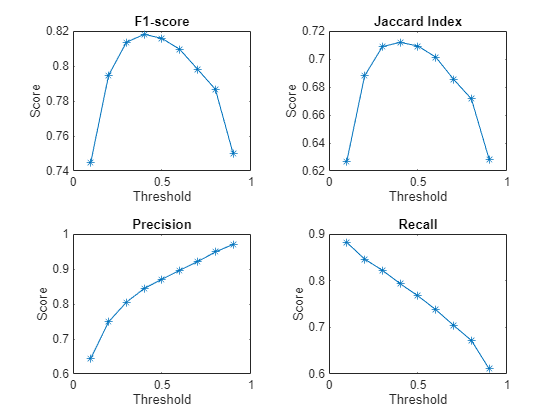### Predict Using New Data

Test the network performance on new images that are not from the COCO data set. The results indicate whether the model can generalize to images from a different underlying distribution.

`imageNames = ["testMultilabelImage1.png" "testMultilabelImage2.png"];`

Predict the labels for each image and view the results.

```figure tiledlayout(1,2) images = []; labels = []; scores =[]; for i = 1:2 img = imread(imageNames(i)); img = imresize(img,inputSize(1:2)); images{i} = img; scoresImg = predict(trainedNet,img)'; YPred = categoriesTrain(scoresImg >= thresholdValue); nexttile imshow(img) title(YPred) labels{i} = YPred; scores{i} = scoresImg; end```#### Investigate Network Predictions

To further explore the network predictions, you can use visualization methods to highlight which area of an image the network is using when making the class predictions. Grad-CAM is a visualization method that uses the gradient of the class scores with respect to the convolutional features determined by the network to understand which parts of the image are most important for each class label. The places where this gradient is large are exactly the places where the final score depends most on the data.

Investigate the first image. The network correctly identifies the cat and couch in this image. However, the network fails to identify the dog.

```imageIdx = 1; testImage = images{imageIdx};```

Generate a table containing the scores for each class.

```tbl = table(categoriesTrain',scores{imageIdx},VariableNames=["Class", "Score"]); disp(tbl)```
``` Class Score ______________ __________ "dog" 0.18477 "cat" 0.88647 "bird" 6.2184e-05 "horse" 0.0020663 "sheep" 0.00015361 "cow" 0.00077924 "bear" 0.0016855 "giraffe" 2.5157e-06 "zebra" 8.097e-05 "elephant" 9.5033e-05 "potted plant" 0.0051868 "couch" 0.80556 ```

The network is confident that this image contains a cat and a couch but less confident that the image contains a dog. Use Grad-CAM to see which parts of the image the network is using for each of the true classes.

```targetClasses = ["dog","cat","couch"]; targetClassesIdx = find(ismember(categoriesTrain,targetClasses));```

Generate the Grad-CAM map for each class label.

```reductionLayer = "sigmoid"; map = gradCAM(trainedNet,testImage,targetClassesIdx,ReductionLayer=reductionLayer);```

Plot the Grad-CAM results as an overlay on the image.

```figure tiledlayout("flow") nexttile imshow(testImage) for i = 1:length(targetClasses) nexttile imshow(testImage) hold on title(targetClasses(i)) imagesc(map(:,:,i),AlphaData=0.5) hold off end colormap jet```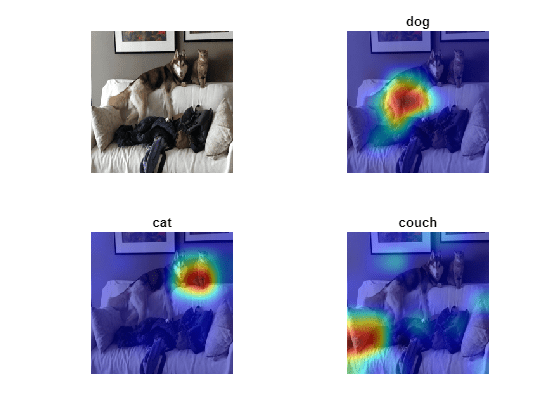The Grad-CAM maps show that the network is correctly identifying the objects in the image.

### Supporting Functions

#### Prepare Data

The supporting function `prepareData` prepares the COCO data for multilabel classification training and prediction.

1. Extract the labels from the file `labelLocation` using the `jsondecode` function.

2. Find the images that belong to the classes of interest.

3. Find the number of unique images. Many images have more than one of the given labels and appear in the image lists for multiple categories.

4. Create the one-hot encoded category labels by comparing the image ID with the lists of image IDs for each category.

5. Combine the data and one-hot encoded labels into a table.

6. Create an augmented image datastore containing the image. Turn grayscale images into RGB images.

The `prepareData` function uses the `COCOImageID` function (attached as a supporting file). To access this function, open this example as a live script.

```function [data, encodedLabel] = prepareData(labelLocation,imageLocation,categoriesTrain,inputSize,doAugmentation) miniBatchSize = 32; % Extract labels. strData = fileread(labelLocation); dataStruct = jsondecode(strData); numClasses = length(categoriesTrain); % Find images that belong to the subset categoriesTrain using % the COCOImageID function, attached as a supporting file. images = cell(numClasses,1); for i=1:numClasses images{i} = COCOImageID(categoriesTrain(i),dataStruct); end % Find the unique images. imageList = [images{:}]; imageList = unique(imageList); numUniqueImages = numel(imageList); % Encode the labels. encodedLabel = zeros(numUniqueImages,numClasses); imgFiles = strings(numUniqueImages,1); for i = 1:numUniqueImages imgID = imageList(i); imgFiles(i) = fullfile(imageLocation + "\" + pad(string(imgID),12,"left","0") + ".jpg"); for j = 1:numClasses if ismember(imgID,images{j}) encodedLabel(i,j) = 1; end end end % Define the image augmentation scheme. imageAugmenter = imageDataAugmenter( ... RandRotation=[-45,45], ... RandXReflection=true); % Store the data in a table. dataTable = table(Size=[numUniqueImages 2], ... VariableTypes=["string" "double"], ... VariableNames=["File_Location" "Labels"]); dataTable.File_Location = imgFiles; dataTable.Labels = encodedLabel; % Create a datastore. Transform grayscale images into RGB. if doAugmentation data = augmentedImageDatastore(inputSize(1:2),dataTable, ... ColorPreprocessing="gray2rgb", ... DataAugmentation=imageAugmenter); else data = augmentedImageDatastore(inputSize(1:2),dataTable, ... ColorPreprocessing="gray2rgb"); end data.MiniBatchSize = miniBatchSize; end```

#### F1-score

The supporting function `F1Score` computes the micro-averaging F1-score .

```function score = F1Score(T,Y) % TP: True Positive % FP: False Positive % TN: True Negative % FN: False Negative TP = sum(T .* Y,"all"); FP = sum(Y,"all")-TP; TN = sum(~T .* ~Y,"all"); FN = sum(~Y,"all")-TN; score = TP/(TP + 0.5*(FP+FN)); end```

#### Jaccard Index

The supporting function `jaccardIndex` computes the Jaccard index, also called intersection over union, as given by

$\frac{|T\cap Y|}{|T\cup Y|}$,

where T and Y correspond to the targets and predictions. The Jaccard index describes the proportion of correct labels compared to the total number of labels.

```function score = jaccardIndex(T,Y) intersection = sum((T.*Y)); union = T+Y; union(union < 0) = 0; union(union > 1) = 1; union = sum(union); % Ensure the accuracy is 1 for instances where a sample does not belong to any class % and the prediction is correct. For example, T = [0 0 0 0] and Y = [0 0 0 0]. noClassIdx = union == 0; intersection(noClassIdx) = 1; union(noClassIdx) = 1; score = mean(intersection./union); end```

#### Precision and Recall

Two common metrics for model assessment are precision (also known as the positive predictive value) and recall (also known as sensitivity).

`$\mathrm{Precision}=\frac{\mathrm{True}\text{\hspace{0.17em}}\mathrm{Positive}}{\mathrm{True}\text{\hspace{0.17em}}\mathrm{Positive}+\mathrm{False}\text{\hspace{0.17em}}\mathrm{Postive}}\text{\hspace{0.17em}}$`

`$\mathrm{Recall}=\frac{\mathrm{True}\text{\hspace{0.17em}}\mathrm{Positive}}{\mathrm{True}\text{\hspace{0.17em}}\mathrm{Positive}+\mathrm{False}\text{\hspace{0.17em}}\mathrm{Negative}}\text{\hspace{0.17em}}$`

The supporting function `performanceMetrics` calculates the micro-average precision and recall values.

```function [precision, recall] = performanceMetrics(T,Y) % TP: True Positive % FP: False Positive % TN: True Negative % FN: False Negative TP = sum(T .* Y,"all"); FP = sum(Y,"all")-TP; TN = sum(~T .* ~Y,"all"); FN = sum(~Y,"all")-TN; precision = TP/(TP+FP); recall = TP/(TP+FN); end```

### References

 Sokolova, Marina, and Guy Lapalme. "A Systematic Analysis of Performance Measures for Classification Tasks." Information Processing & Management 45, no. 4 (2009): 427–437.